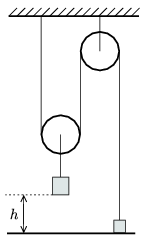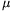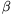Mathematical and Physical Journal
for High Schools
Issued by the MATFUND Foundation
 Already signed up? New to KöMaL?

# KöMaL Problems in Physics, September 2007

Show/hide problems of signs:## Problems with sign 'M'

Deadline expired on October 11, 2007.

M. 280. Drop some oil to the surface of water. Plot the graph of the diameter of the patch of oil as a function of the quantity of the dropped oil.

What is the width of the oil layer spread out on the surface of water?

Examine the phenomenon at different temperatures.

(6 pont)

statistics## Problems with sign 'P'

Deadline expired on October 11, 2007.

P. 3993. Pour some soda water or soft drink to a glass and drop some raisins into it.

Observe what happens in the next few minutes.

(3 pont)

statistics

P. 3994. A 60 kg boy notices a nice fruit on a tree. Even with stretched arms he cannot reach it, he is a 0.5 m below the fruit. The boy crouches down - his centre of mass descends 0.4 m - and then he leaps up. Thus he just reaches the fruit. Assume that he exerts a constant force on the ground while he touches it.

a) What is this force at least?

b) What is the average power of the boy?

(4 pont)

statistics

P. 3995. A 2 kg lead ball and a big medicine ball of mass 2 kg are dropped from the same height. The lead ball reaches the ground in 2 seconds and the medicine ball hits the ground with a speed of 10 m/s.

Calculate the work done on the medicine ball by the air drag.

(4 pont)

statistics

P. 3996. A courier runs from the end of an 80 m long marching troop to its front, and then it runs back to the end with the same speed. During this the marching troop covers a distance of 150 m. Calculate the distance covered by the courier.

(4 pont)

statistics

P. 3997. The mass of the body which is hanging on the rope attached to the movable pulley is four times as much as the mass of the body which is fixed to the ground. At a given instant the fixed body is released. To what height does it ascend? (The mass of the pulleys and the ropes is negligible.)(4 pont)

statistics

P. 3998. A cylinder with a piston in it encloses some air. Initially the volume of the air is V=0.1 m3, its temperature is 27 oC, and its pressure is p=113 kPa. The system is operated as a heat engine, while the air is taken through a cyclic process which consists of two isobaric processes at pressures of p and 2p and two isochoric processes at volumes of V and 3V.

a) What is the work done by the gas in the cyclic process?

b) What is the efficiency of this heat engine?

(4 pont)

statistics

P. 3999. Explain the formation of frost and freezing rain.

(3 pont)

statistics

P. 4000. A coil is connected to an alternating emf of voltage 24 V and of frequency 50 Hz. The reading on the ammeter connected to the coil in series is 10 mA. If a 1F capacitor is connected to the coil in series the ammeter shows 10 mA again.

What would be the reading on the ammeter if the coil was connected to a 24 V dc voltage supply?

(4 pont)

statistics

P. 4001. Two objects are placed to the principal axis of a thin converging lens. One is 20 cm from the lens and the other is on the other side of the lens at a distance of 40 cm from the lens. The images of both objects are in the same plane. What is the focal length of the lens?

(4 pont)

statistics

P. 4002. The starting isotope of a decay chain is 238U and the stable final isotope of this decay chain is 206Pb. How manydecays, and how manydecays occur in the decay chain?

(3 pont)

statistics